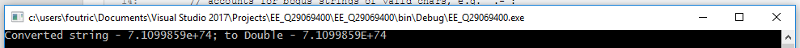Atof Equivalent in C#

Is it possible to convert this code to C#?

``````	int pos=0,p=0;
double v1,expo,res;

p=value.find("e+");

if(value.find("e+")!=-1 ||value.find("e-")!=-1)
{
pos=value.find("e");
if(atof(value.substr(0,pos).c_str()))
{
v1=StringToDouble(value.substr(0,pos));
expo=StringToDouble(value.substr(pos+1,(value.length()-(pos+1))));
res=pow(10,expo)*v1;
return DoubleToString(res);

}
}
``````

I saw this online, but wasn't sure how to get the Takewhile function to work.
https://msdn.microsoft.com/en-us/library/system.linq.enumerable.takewhile(v=vs.100).aspx

``````float myAtof(string myString)
{
Predicate<char> testChar = c => c == '.' ||
c == '-' ||
c == '+' ||
char.IsDigit(c);

myString = new string(myString.Trim().TakeWhile(testChar).ToArray());

if (myString.Length > 0)
{
float rvl;

// accounts for bogus strings of valid chars, e.g. ".-":
if (float.TryParse(myString, out rvl))
{
return rvl;
}
}

return 0;
}
``````
Who is Participating?
I wear a lot of hats...

"The solutions and answers provided on Experts Exchange have been extremely helpful to me over the last few years. I wear a lot of hats - Developer, Database Administrator, Help Desk, etc., so I know a lot of things but not a lot about one thing. Experts Exchange gives me answers from people who do know a lot about one thing, in a easy to use platform." -Todd S.

DeveloperCommented:
In C#, we use the inbuilt Convert Methods, e.g. -
``````using System;

namespace EE_Q29069400
{
class Program
{
static void Main(string[] args)
{
string factorial = "7.1099859e+74";
double converted = Convert.ToDouble(factorial);
Console.WriteLine(\$"Converted string - {factorial}; to Double - {converted}");
}
}
}
``````
You can also use the type specific Parse and/or TryParse methods; e.g. -
``````using System;

namespace EE_Q29069400
{
class Program
{
static void Main(string[] args)
{
string factorial = "7.1099859e+74";
double converted;
if (double.TryParse(factorial, out converted))
{
Console.WriteLine(\$"Converted string - {factorial}; to Double - {converted}");
}
else
{
Console.WriteLine(\$"Could not convert {factorial}");
}
}
}
}
``````

Each of the preceeding produce the following output --saige-

Experts Exchange Solution brought to you by